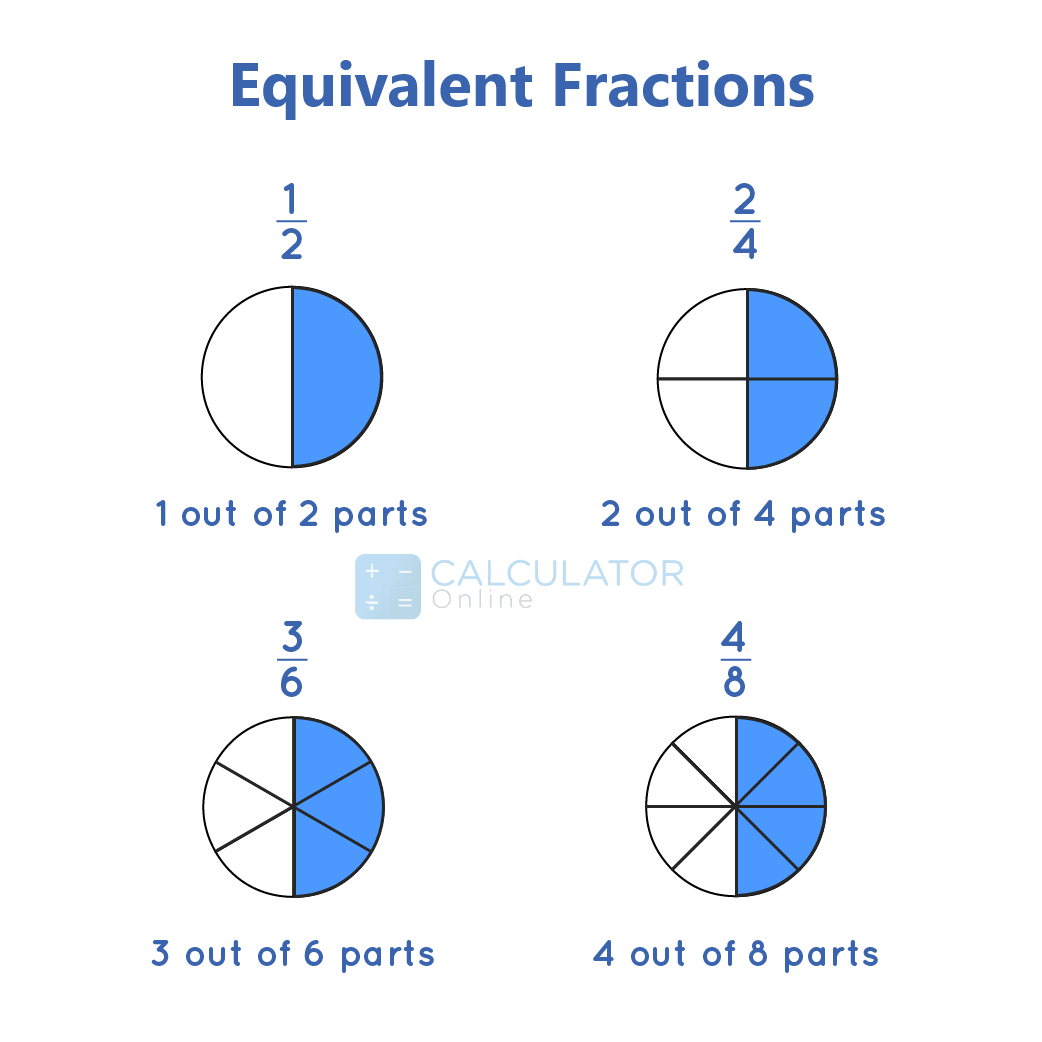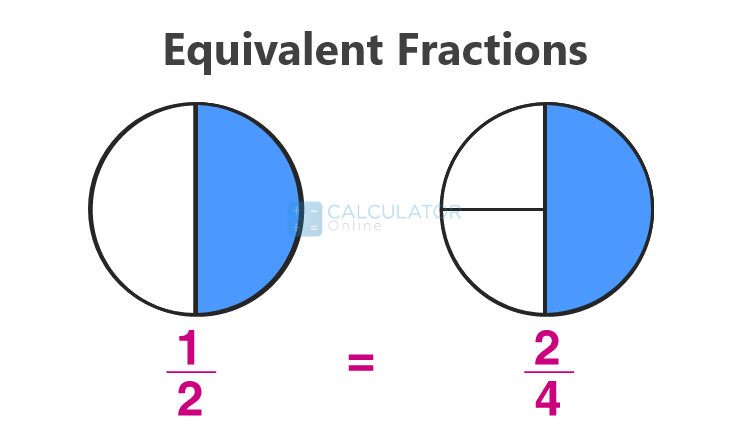• Hire UsUh Oh! It seems you’re using an Ad blocker!

We always struggled to serve you with the best online calculations, thus, there's a humble request to either disable the AD blocker or go with premium plans to use the AD-Free version for calculators.

Or# Equivalent Fractions

## What is equivalent fraction?

In mathematical form, equivalent fractions can be defined as:

“Two or more fractions that have different numerators and denominators but are equal when reduces into simplest form”

Let’s suppose a/b and c/d are two fractions. When these fractions are simplified, the given result comes in equivalent fractions, say e/f, then we call them equal to each other.
Whether you want to determine which fraction is equivalent to or not, you can use this Equivalent fractions calculator to perform calculations swiftly.### Equivalent fractions examples:

Consider the fraction of 3/9 is 1/3
Consider the fraction of 4/8 is 1/2
Let’s consider a mixed number 1 ½. Calculates its equivalent fraction.
Given:
Mixed fraction = 1 ½
Solution:
Firstly, convert mixed fraction into an improper form.

1 ½ = (1 × 2 + 1)/2 = (2 + 1)/2 = 3/2

The equivalent fractions for the above fraction can be calculated as: :

3/2 = (3 × 1)/(2 × 1) = 3/2

3/2 = (3 × 2)/(2 × 2) = 6/4

3/2 = (3 × 3)/(2 × 3) = 9/6 and so on.
However, you can carry out calculations regarding to arithmetic operations of add (+), subtract (-), multiply (×), and divide (÷) by using an online mixed number calculator smoothly

### Methods to Determine Equivalent Fractions

Let’s discuss simply three methods to calculate the value of identical fractions and also determine that two fractions are equivalent or not.

#### Method 1: Make the Denominators the same

Two fractions are equivalent if the denominators has the same value.
Example:
Find out if 2/4 and 4/8 are equivalent.

Taking the LCM of 4 and 8 = 8

Multiply 2/4 by 2/2 to make the denominator equal to 8.

2/4 × 2/2 = 4/8

Therefore, by making the denominators the same. We can say that 2/4 and 4/8 are equivalent fractions.

#### Method 2: Cross Multiply

If both the products are the same in the fraction by cross multiplication, we can say that the fractions are the equivalent.
Example:
Two fractions are given above such as 1/2 and 3/6
Take cross multiple both the fractions to get:
= 1 x 6 = 6

= 2 x 3 = 6
Hence, both the values are equal so we can say that 1/2 and 3/6 are equivalent fractions.
If you want more calculations related to the cross multiplications, you can use this free-source online cross multiply calculator

#### Method 3: Convert to decimals

We can simply determine decimals if two fractions are given to check out they are equivalent  fractions.
Example:
Consider two fractions 1/4 and 3/12, then convert them in decimal form.
1/4 = 0.25
3/12 = 0.25
Hence, both the fractions have the same decimal so they are equivalent.

### Equivalent fractions chart

Let’s have a look at some comparable fractions that clarify your concept about equivalent fractions.
 Fraction form Equivalent fraction 2/3 4/6, 6/9, 8/12, 10/15 … 1/4 2/8, 3/12, 4/16, 5/20 … 1/2 2/4, 3/6, 4/8, 5/10 … 1/3 3/9, 4/12, 5/15 … 1/5 2/10, 3/15, 4/20, 5/25 … 2/4 4/8, 6/12, 8/16, 10/20 … 6/9 12/18, 18/27, 24/ 36 … 1/6 2/12, 3/18, 4/24 … 5/10 10/20, 15/30, 20/40 … 3/4 6/8, 9/12, 12/16, 15/20 … 3/6 6/12, 9/24, 12/24 …
Note: For adding, subtracting, multiplying, and dividing 2 or 3 fractions, an online fraction calculator is available to proceed calculation instantly.

### How to find equivalent fractions?

Generally, comparable fractions are estimated by multiplying or dividing the numerator and the denominator with the same number. Look at the given examples to explore your concept and verify your answer.
Example #1:

Let’s take the fractions 1/6 and find its equivalent fractions by multiplying the same number.
Given:

Fraction = 1/6
Solution:

Multiply the given fraction with 3 to get 1/6 × 3/3 = 4/18
Again multiply the fraction with 4 to get 1/6 × 4/4 = 4/24
Then again multiply the fraction with 5 to get 1/6 × 5/5 = 5/30
Hence, 1/6 = 4/18 = 4/24 = 5/3

Example #2:

Find the equivalent fraction of 36/54 by dividing the same number.
Given:

Fraction of 36/54
Solution:

Since 2 is a common factor of 36 and 54. So, 36/54=
36÷2/54÷2 = 18/27
Now again, 3 is a common factor of 18 and 27. So, 18/27=
18÷3/27÷3= 6/9
Again, 3 is a common factor of 6 and 9.So, 6/9=
6÷3/9÷3 = 2/3
Hence, the given result as: 36/54 = 18/27 = 6/9 =  2/3